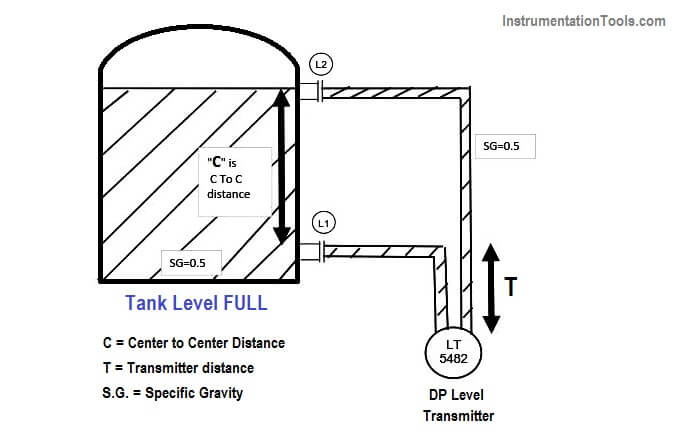# How to Find LRV and URV of Closed Tank DP Level Transmitter

Find out the configuration of Lower Range Value (LRV) and Upper Range Value (URV) of a Differential Pressure (DP) Level Transmitter. Every DP transmitter needs to be configured using a HART communicator.

Now let us see the formula for calculating the LRV and URV of the transmitter.

This formula needs two values only

S.G – (Specific gravity which has no unit so no tension of compatible units, if the process has given you density it can easily be converted into SG )

Length – All lengths must be entered in the formula as INCHES only !!

The Formula is Pressure(Head) = Specific Gravity * Height

and TADA !! you have answer in inH2O !!

## As we are using S.G which means comparing the density with that of water and the height is in inches. Hence when we multiply the two we get the answer in Inches of water column (no matter what the fluid is answer will be in inH2O ).Given:- The C to C Distance is 1000 inches. (center to center)

The Transmitter is 50 inches below lower taping

## At 4 mA Condition (LRV)

HP = “ 0.5 * 0 (As at 4 mA no liquid is inside the tank) “ +  0.5 * 50 inches (as transmitter is 50 inches below nozzle)

HP=25 Inches of Water Column is the pressure experienced at HP tap

LP  = “ 0.5 * 1000 (As the wet leg impulse line is fill with fluid ) “ + 0.5 * 50inches (as transmitter is 50 inches below nozzle)

LP = 500+25=525 Inches of Water Column is the pressure experienced at LP tap

Output =HP-LP= 25-525=-500 inches of water column must be calibrated for 4 mA

Therefore 4mA =(-500) InWC

so, lower range value (LRV) to be configured in Transmitter is -500 InWC

## At 20 mA Condition (URV)HP =  0.5 * 1000 (As at 20 mA liquid will be filled in the entire range) +

0.5 * 50inches (as the transmitter is 50 inches below nozzle)

HP= 500+25=525 Inches of Water Column is the pressure experienced at HP tap

LP = “ 0.5 * 1000 (As the wet leg impulse line is filled with fluid inside the tank ) “  +

0.5 * 50inches (as the transmitter is 50 inches below nozzle)

LP = 500+25=525 Inches of Water Column is the pressure experienced at LP tap

Output = HP-LP=525-525=-0 inches of water column must be calibrated for 20 mA

Therefore 20mA = 0 InWC

so, upper range value (URV) to be configured in the Transmitter is 0 InWC

The calibration Range will be -500 to 0 inches of the water column.

REFERENCE:- This above formula is taken from Emerson and to date I have found this to be the easiest and simplified literature explaining the level calculation.

#### Author: Asad Shaikh

Don't Miss Our Updates
Be the first to get exclusive content straight to your email.
We promise not to spam you. You can unsubscribe at any time.
1.